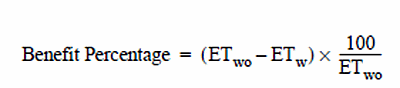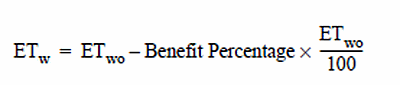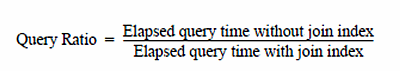# Cost/Benefit Analysis for Join Indexes | Teradata Vantage - 17.10 - Cost/Benefit Analysis for Join Indexes - Advanced SQL Engine - Teradata Database

## Teradata Vantage™ - Database Design

Product
Release Number
17.10
Published
July 2021
Language
English (United States)
Last Update
2021-07-27
dita:mapPath
kko1591750222108.ditamap
dita:ditavalPath
kko1591750222108.ditaval
This topic introduces the concepts of cost/benefit analyses for various types of join indexes. Several different computations are described to support these analyses including the following metrics.
• Cost
• Benefit
• Benefit percentage
• Payback
• Query ratio

## Join Index Benefits Summary

The benefits of join indexes vary considerably among the various types of joins used as well as between simple and aggregate types. The following bulleted list summarizes the conclusions to be drawn from the performance analyses performed.
• All queries that require join processing benefit from join indexes; often dramatically, and sometimes spectacularly.
• In all tests performed, aggregate join indexes strongly outperform simple join indexes, both with respect to their performance and with respect to their maintenance burden. Queries using an aggregate join index frequently run hundreds of times faster than the same queries against the same tables when no join index is defined.
• The benefits of simple join indexes are typically more modest. Queries using a simple join index typically run from 1.3 to 4.9 times faster than the same queries against the same tables when no join index is defined.
• In-place join indexes handily outperform any join indexes that redistribute rows.

## Computing the Benefits of Join Indexes

This topic describes a measure you can use to calculate the benefit of a join index.

Begin the calculation by computing some preliminary measures, as described in the following set of equations.

Define the benefit of a join index as follows:

Benefit = ET wo– ET w

where:

Syntax element … Specifies elapsed query time …
ET wo without a join index defined for the query.
ET w with a join index defined for the query.

Benefit is a simple measure that measures the advantage of a query in terms of the difference between its elapsed completion time when a join index is not defined and its elapsed completion time when a join index is defined.

Define the benefit percentage as follows:The benefit percentage for a query is just a normalized form of the raw benefit. It provides an easily understood measure of the reduction in processing time gained by creating the join index.

Rearrange terms to determine the elapsed time to process a query with the join index defined. This measure is necessary to determine if the cost of creating the join index exceeds its usefulness in reducing response time.## Computing the Query Ratio

Define the query ratio as follows:The query ratio is a normalized measure of how much faster a query executes with a join index than without a join index. For example, consulting the table about Creation and Elapsed Query Times for Different Join Indexes, you find a column of calculated query ratios.

You interpret these ratios as follows. Consider the query ratio for the creation cost of a single-table aggregate join index. The reported query value for this join index is 41. This means that the test query runs 41 times faster with the single-table aggregate join index defined than it does without it.

## Computing the Payback Factor

This topic explains how to calculate the payback factor for a join index. Note that the term Costs is something you must measure. It is the time required to compute the join index being investigated.

Payback Factor = Costs ÷ Benefit

where:

Syntax element … Specifies …
Costs the processing time required to create the join index.

Because the term payback factor represents a number of queries, it is always rounded up to represent a whole number. The smaller the payback factor, the sooner benefits accrue from using the join index.

## Example of Computing a Payback Factor for a Simple Join Index

Suppose you have a frequently performed, non-aggregate join query that you think might benefit from a join index. You decide to test the join index and collect the following data.

Parameter Measured Value (seconds)
ET wo                                           416
ET w                                           226
Cost                                         1055

What is the payback factor for creating this join index?

Benefit = 416 – 226 = 190 seconds

Benefit Percentage = 190 × (100 ÷ 416) = 46%

The interpretation of the benefit percentage is that the time to complete the query is cut nearly in half.

Payback factor = 1055 ÷ 190 = 6

The interpretation of the payback factor is that the cost of creating the join index is recovered if the query is run six or more times.

## Two More Brief Examples

The following examples work through the following join indexes.
• In-place simple join index
• Ad hoc aggregate join index

Follow the identical procedure to that used in the detailed example.

For the in-place simple join index, the calculation is as follows.To recover its cost in a 30-day period, this query must be run slightly more than once per business day.

For the ad hoc aggregate join index, the calculation is as follows.To recover its cost in a 30-day period, this query must be run once per week.